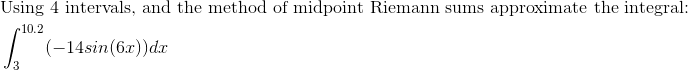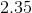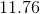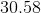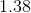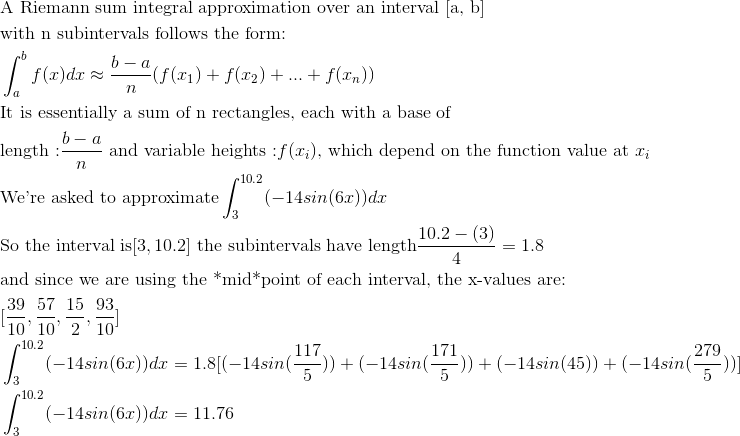Calculus 2 : Introduction to Integrals

Example Questions

Example Question #1831 : Calculus Ii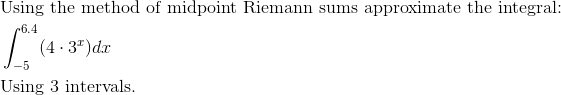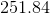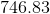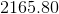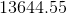Explanation: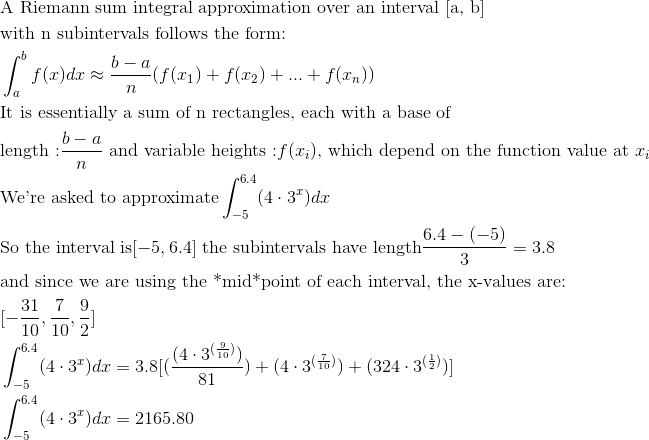Example Question #1832 : Calculus Ii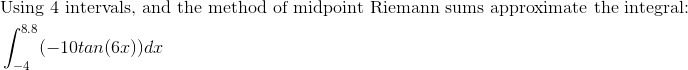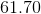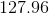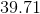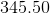Explanation: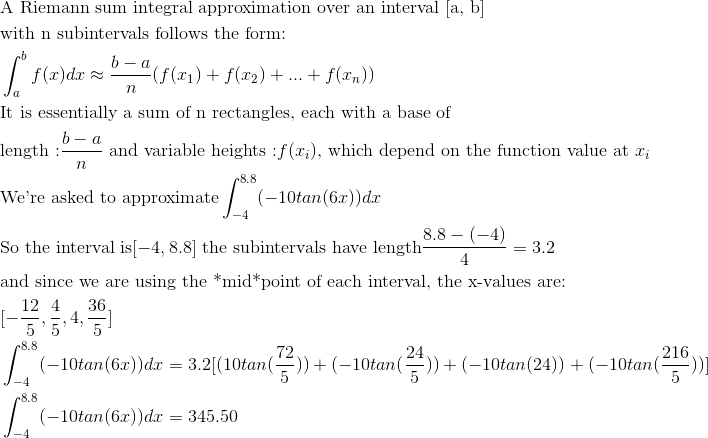Example Question #1833 : Calculus Ii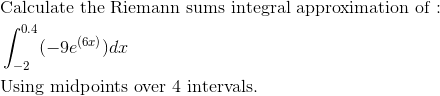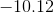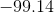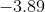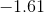Explanation: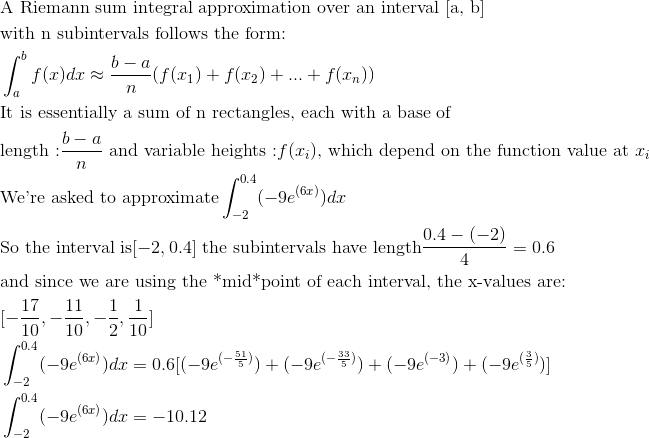Example Question #1834 : Calculus Ii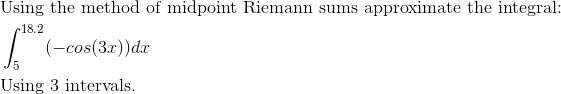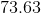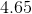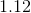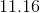Explanation: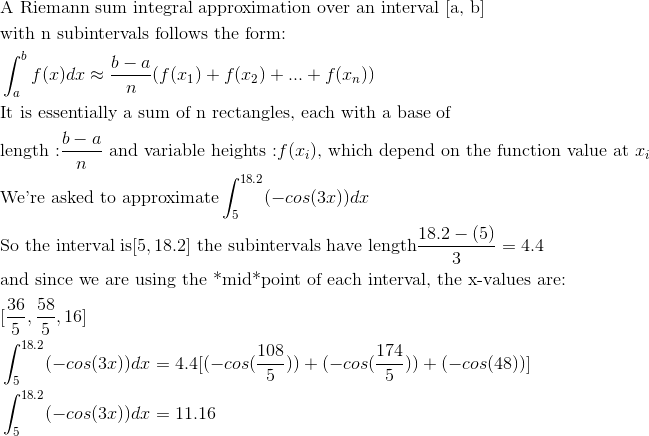Example Question #1835 : Calculus Ii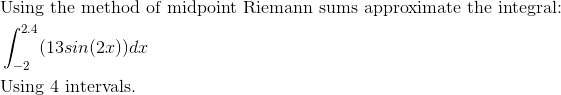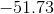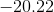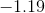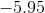Explanation: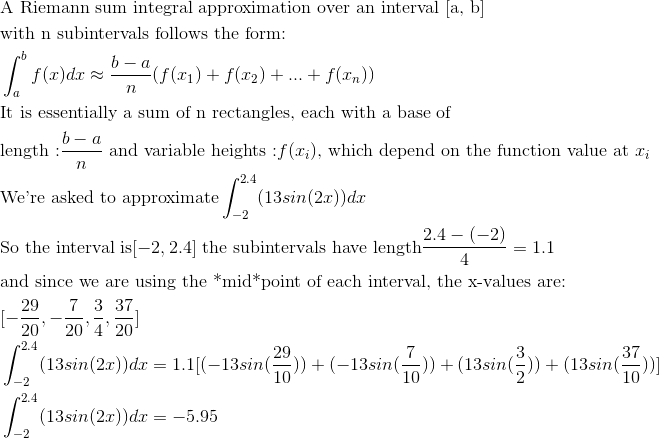Example Question #1836 : Calculus Ii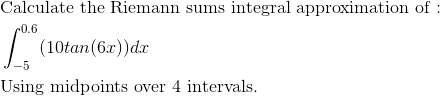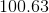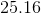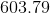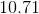Explanation:Example Question #1837 : Calculus Ii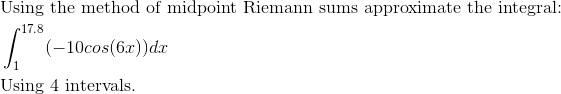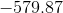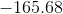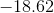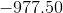Explanation: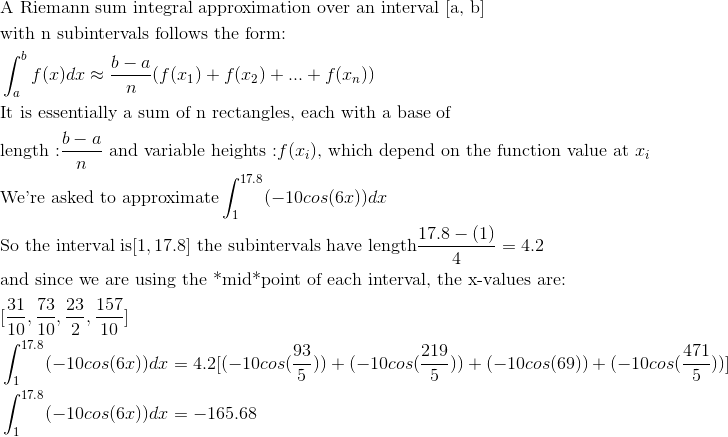Example Question #1838 : Calculus Ii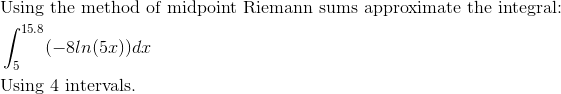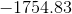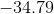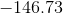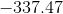Explanation: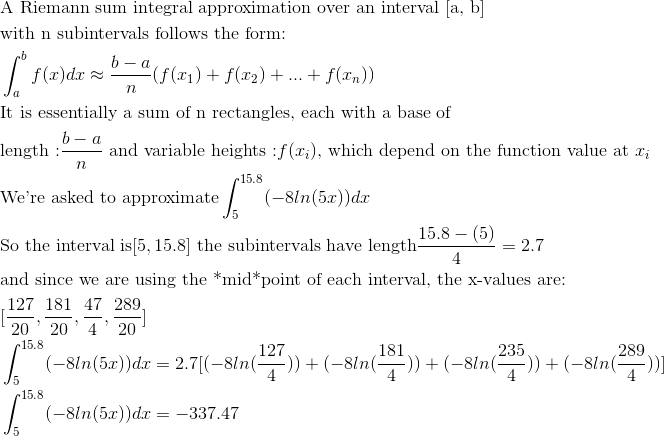Example Question #1839 : Calculus Ii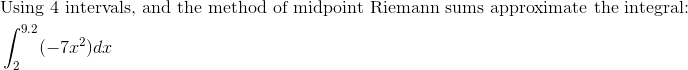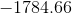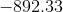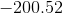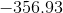Explanation: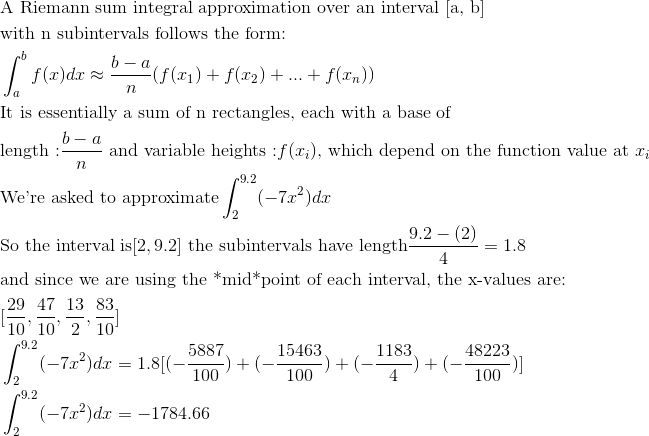Example Question #41 : Riemann Sums